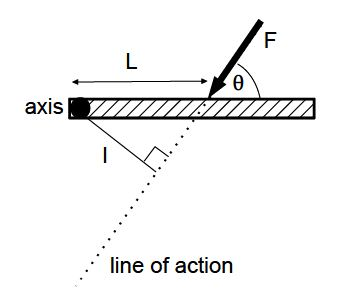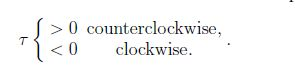Physics

# What is Mechanical Equilibrium?

We consider a meter stick that is free to rotate about a fixed axis (at the end). Intuition tells us that the applied force F is more “effective” the greater the distance L from the axis, and the greater the angle θ < 90°. For a quantitative definition, we draw a straight line through the force called the line of action. We then draw a line perpendicular to the line of action through the axis: the distance between the axis and the line of action is the lever arm,l = L sin θ.

The magnitude of the torque is then

׀τ  ׀= Fl = FL sinθ.

The torque is a vector quantity. For rotation about a fixed axis, the torque is either positive or negative for counter-clockwise- and clockwise rotation, respectively,The unit of torque is force times distance, [τ] = [F] * [l] = N * m. Note that N * m = J, but this is not used since torque and work are different physical quantities.

For a point mass, the condition ∑ F = 0 is sufficient to guarantee that an object initially at rest, stays in rest. For an extended object, the condition ∑ F = 0 only guarantees that there there is no translational motion, i.e., motion to the left and right, up and down, and into- and out-of the page. However, the object could still rotate. In general, the torque depends of the axis of rotation. In the case when the net force is zero, ∑ F = 0, the torque is independent of the choice of the axis. It follows that the condition ∑ τ = 0 is independent of the choice of the axis of rotation. We thus have the condition for mechanical equilibrium,

∑ F= 0 and ∑ τ = 0 (mechanical equilibrium)

At the introductory physics level, we solve restricted problems where the axis of rotation is out-of or into- the page, and all forces are in the page’s plane. In this case, the condition for mechanical equilibrium consists of three equations so that we have at most three unknown quantities. Typical cases are:

(1) three unknown forces in known directions [e.g., along a cable], and

(2) one unknown force in a known direction and one unknown force in an unknown direction. Many practical problems are thus outside the scope of introductory physics; we cannot find the forces in each of the four legs of a table when an object sits on it in an arbitrary location.

In many cases, the equation for the net torque ∑ τ = 0 can be simplified by making a “smart” choice of the axis. For example, the lever arm for one or two forces is zero and the corresponding forces do not contribute to the net torque.